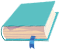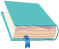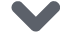# 使用skimage处理图像数据的9个技巧｜视觉进阶

### 概述

• Python中的skimage包可以快速入门图像处理

• 学习使用skimage进行图像处理的8个强大技巧

• 每个skimage的技巧都附加了Python代码

### 目录

1. 什么是skimage?为什么要使用它?

2. 使用skimage在Python中读取图像

3. 调整图像大小

4. 上下翻转图像

5. 旋转不同角度

6. 水平和垂直翻转

7. 图像裁剪

8. 改变图像亮度

9. 使用滤镜

### 什么是skimage?为什么要使用它?

Python中有多个库和框架可让我们处理图像数据。那么,为什么要使用skimage?在深入研究本文之前,让我在这里回答。

Scikit-image或skimage是一个用于图像预处理的开源Python包。

### 1.使用skimage读取图像

#### 使用skimage加载图像

from skimage.io import imread, imshowfrom skimage import dataimage = data.astronaut()imshow(image)

### 使用skimage从系统读取图像

from skimage.io import imread, imshowimport matplotlib.pyplot as plt%matplotlib inlineimage_gray = imread('images.jpeg', as_gray=True)imshow(image_gray)

image_gray = imread('images.jpeg', as_gray=True)print(image_gray.shape)print(image_gray)
(258,195)[[0.73586314 0.77115725 0.7907651 ... 0.11822745 0.11822745 0.11430588] [0.65743176 0.70056902 0.72017686 ... 0.11822745 0.11430588 0.11430588] [0.41401176 0.45714902 0.48067843 ... 0.11430588 0.11430588 0.11038431] ... [0.73491725 0.73491725 0.73491725 ... 0.42055725 0.42055725 0.42055725] [0.72594314 0.72986471 0.72986471 ... 0.41750667 0.41750667 0.41750667] [0.72594314 0.72986471 0.72986471 ... 0.41750667 0.41750667 0.41750667]

from skimage.io import imread, imshowimport matplotlib.pyplot as plt%matplotlib inlineimage_color = imread('images.jpeg', as_gray=False)print(image_color.shape)imshow(image_color)
(258,195,3)

### 2.更改图像格式

from skimage.color import rgb2grayimg = imread('images.jpeg')img_new = rgb2gray(img)plt.subplot(121), imshow(img)plt.title('RGB Format') plt.subplot(122), imshow(img_new)plt.title('Grayscale Format') plt.show()

• 色调(Hue)是色轮上的度数,其中0代表红色,120代表绿色,240代表蓝色,360再次代表红色。

• 饱和度(Saturation)表示该颜色的百分比,其中0是白色,而100是全色。

• 明度(Value)表示不同数量的黑色或白色混合。

• 亮度(Lightness)是显示图像阴影的另一种方式,其中0为黑色,而1为白色。

from skimage.color import rgb2hsvimg = imread('images.jpeg')img_new = rgb2hsv(img)plt.subplot(121), imshow(img)plt.title('RGB Format') plt.subplot(122), imshow(img_new)plt.title('HSV Format') plt.show()

### 3.使用skimage调整图像大小

from skimage.transform import resizeimg = imread('images.jpeg')#缩放图片img_resized = resize(img, (300, 300))#显示图片plt.subplot(121), imshow(img)plt.title('Original Image')plt.subplot(122), imshow(img_resized)plt.title('Resized Image')plt.show()

### 4.使用skimage重新缩放(放大/缩小)图片

from skimage.transform import rescaleimg = imread('images.jpeg')img_rescaled = rescale(img, scale=(0.5, 0.5))plt.subplot(121), imshow(img)plt.title('Original Image')plt.subplot(122), imshow(img_rescaled)plt.title('Rescaled Image')plt.show()

### 5.使用skimage以不同角度旋转图像

from skimage.transform import rotateimage = imread('tilt_image.png')image_rotated = rotate(image, angle=45)imshow(image_rotated)

from skimage.transform import rotateimage = imread('tilt_image.png')image_rotated = rotate(image, angle=45, resize=True)imshow(image_rotated)

### 6.水平和垂直翻转图像

NumPy提供flipudfliplr函数分别用于在水平和垂直轴上翻转图像。

from numpy import fliplr, flipuddog = imread('Puppy.jpg')cat = imread('whiskers.jpg')dog_flip = fliplr(dog)cat_flip = fliplr(cat)plt.subplot(141), imshow(dog)plt.subplot(142), imshow(dog_flip)plt.subplot(143), imshow(cat)plt.subplot(144), imshow(cat_flip)plt.show()

### 7.裁剪图像

• 首先，简单地指定你想要的新图像形状。在本例中，它是image[100:967, 100:1500]。这对于单个图像来说很好。如果我们有多个图像呢?我们不得不为每个图像都提到新的图像形状(不是很友好)。

• 另一种方法是使用当前图像形状计算裁剪后的图像尺寸，可以使用image.shape命令来确定。所以新图像的高度是[100:img.shape-100] ，宽度为 [100:img.shape-100].

image = imread('warriors.jpg')# 只选择图像的一部分cropped = image[100:(img.shape-100),100:(img.shape-100)]plt.subplot(121), imshow(image)plt.title('Original Image')plt.subplot(122),imshow(cropped)plt.title('Cropped Image')plt.show()

### 8.使用skimage更改图像亮度

from skimage import exposure#调节亮度image = imread('images.jpeg')image_bright = exposure.adjust_gamma(image, gamma=0.5,gain=1)image_dark = exposure.adjust_gamma(image, gamma=1.5,gain=1)# 显示图像plt.subplot(131), imshow(image)plt.title('Original Image')plt.subplot(132),imshow(image_bright)plt.title('Bright Image')plt.subplot(133),imshow(image_dark)plt.title('Dark Image')plt.show()

### 9.在skimage中使用滤镜

from skimage.filters import medianimage = imread('images.jpeg', as_gray=True)image_median = median(image)# 显示图像plt.subplot(121), imshow(image)plt.title('Original Image')plt.subplot(122),imshow(image_median)plt.title('Smooth Image')plt.show()

from skimage.filters import sobel_himage = imread('images.jpeg', as_gray=True)image_sobelh = sobel_h(image)# 显示图像plt.subplot(121), imshow(image)plt.title('Original Image')plt.subplot(122),imshow(image_sobelh, cmap = True)plt.title('Horizontal Edge')plt.show()

### 结尾

欢迎扫码关注磐创AI点击下方 |  | 了解更多